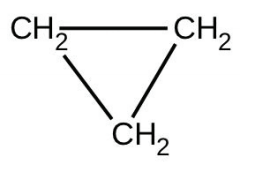# Problem: There are two molecules with the formula C3H6. Propene, CH3CH=CH2 , is the monomer of the polymer polypropylene, which is used for indoor-outdoor carpets. Cyclopropane is used as an anesthetic:When heated to 499 °C, cyclopropane rearranges (isomerizes) and forms propene with a rate constant of 5.95 × 10−4 s −1. What is the half-life of this reaction? What fraction of the cyclopropane remains after 0.75 h at 499 °C?

###### FREE Expert Solution
96% (259 ratings)
###### Problem Details

There are two molecules with the formula C3H6. Propene, CH3CH=CH2 , is the monomer of the polymer polypropylene, which is used for indoor-outdoor carpets. Cyclopropane is used as an anesthetic:When heated to 499 °C, cyclopropane rearranges (isomerizes) and forms propene with a rate constant of 5.95 × 10−4 s −1. What is the half-life of this reaction? What fraction of the cyclopropane remains after 0.75 h at 499 °C?